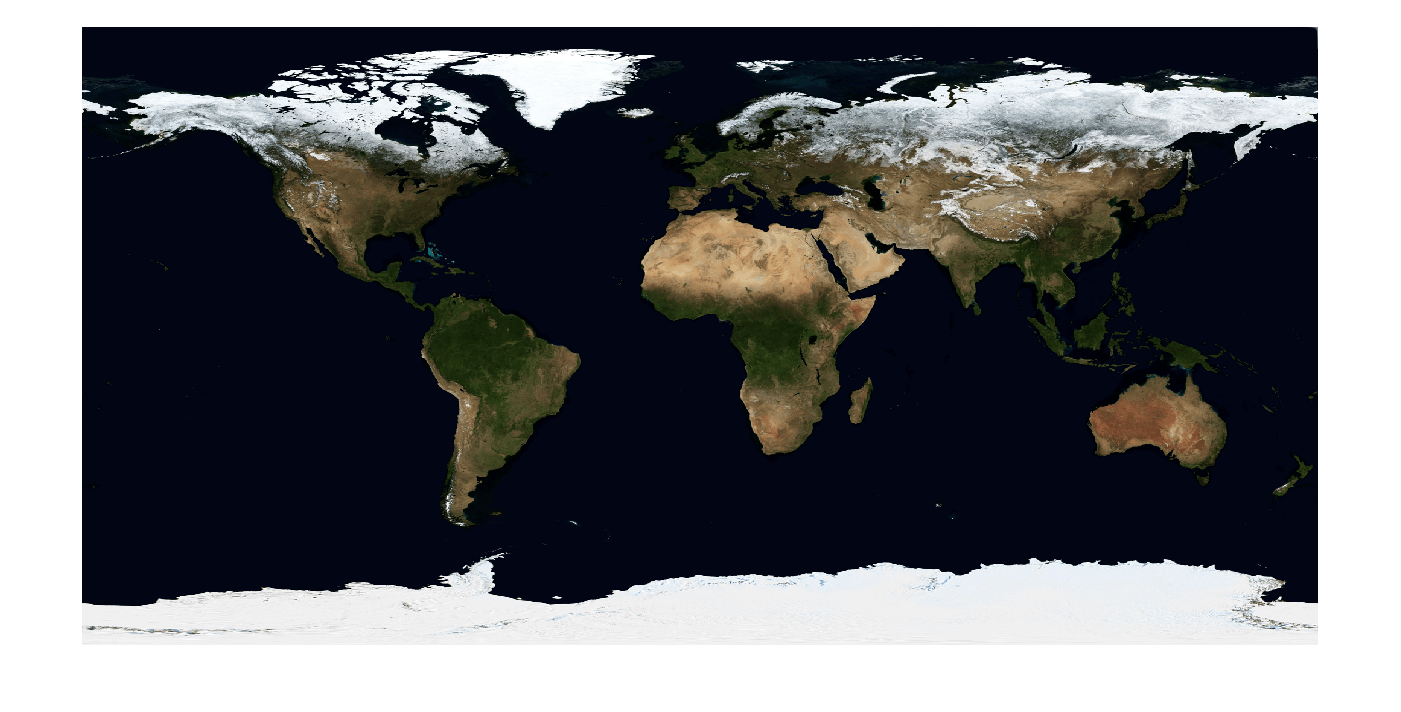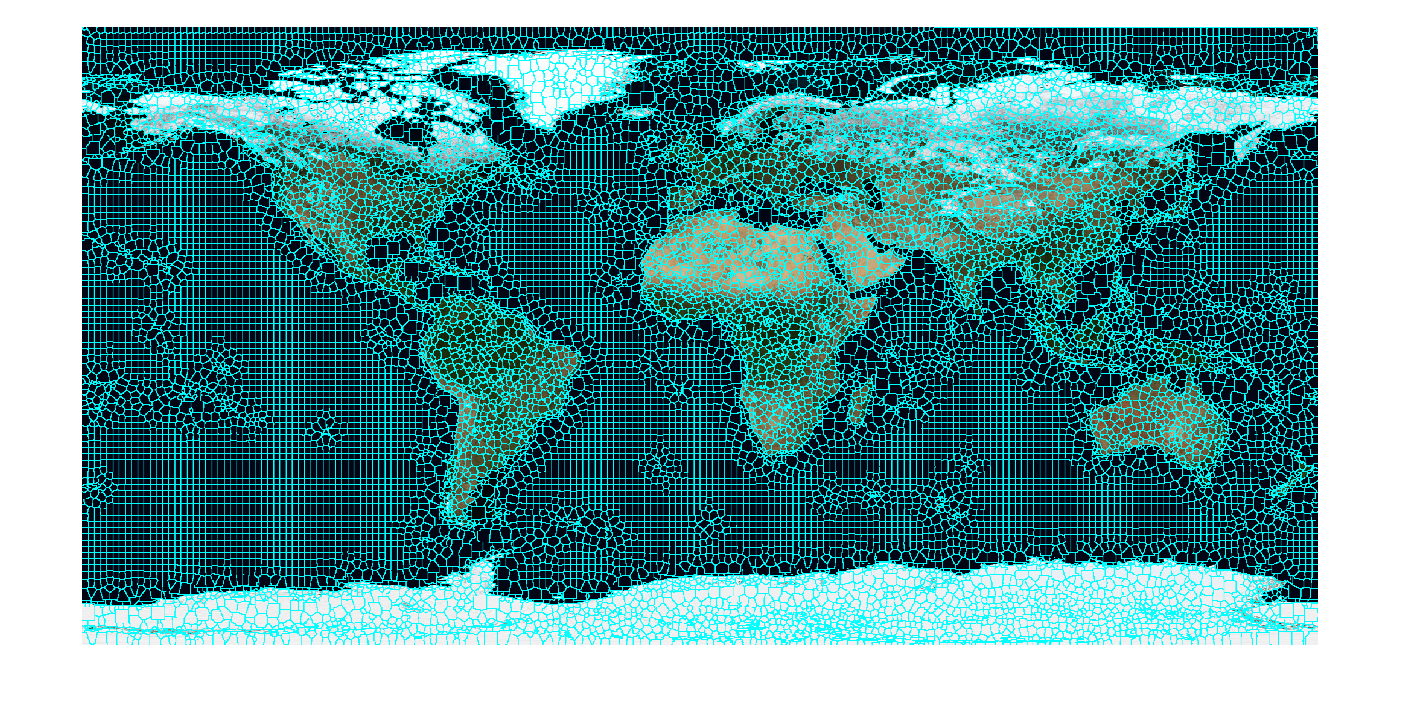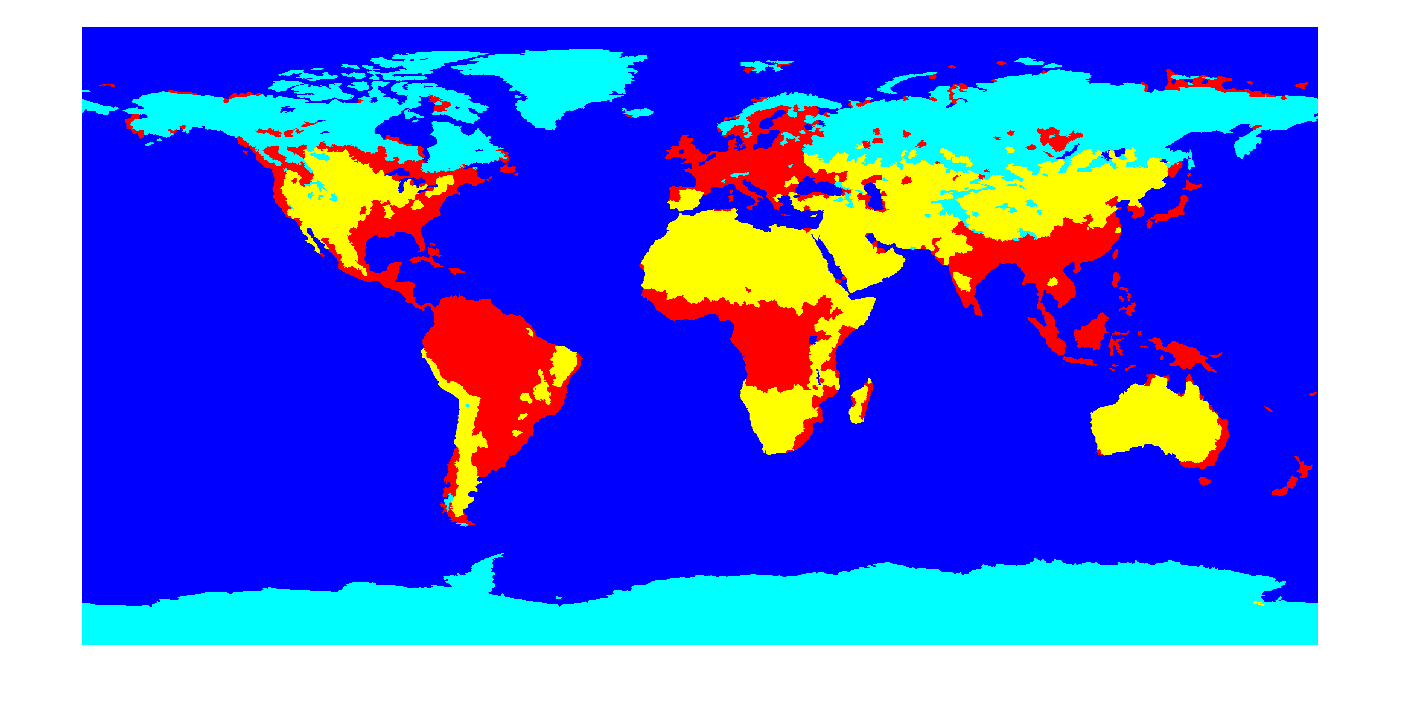# Plot Land Classification with Color Features and Superpixels

This example shows how to perform land type classification based on color features using K-means clustering and superpixels. Superpixels can be a very useful technique when performing segmentation and classification, especially when working with large images. Superpixels enable you to break an image into a set of structurally meaningful regions, where the boundaries of each region take into account edge information in the original image. Once you break an image into superpixel regions, classification algorithms can be used to classify each region, rather than having to solve the classification problem over the full original image grid. The use of superpixels can provide large performance advantages in solving image classification problems while also providing a high quality segmentation result.

Read an image into the workspace. For better performance, this example reduces the size of the image by half. Visually, there are four types of land that are distinguishable in the blue marble image based only on color features: forested regions, dry/desert regions, ice covered regions, and water.

```url = "https://eoimages.gsfc.nasa.gov/images/imagerecords/74000/74192/" ... + "world.200411.3x5400x2700.jpg"; A = imread(url); A = imresize(A,0.5); imshow(A)```Convert the image to the L*a*b* color space.

`Alab = rgb2lab(A);`

Compute the superpixel oversegmentation of the original image and display it.

```[L,N] = superpixels(Alab,20000,isInputLab=true); BW = boundarymask(L); imshow(imoverlay(A,BW,"cyan"))```Create a cell array of the set of pixels in each region.

`pixelIdxList = label2idx(L);`

Determine the median color of each superpixel region in the L*a*b* color space.

```[m,n] = size(L); meanColor = zeros(m,n,3,"single"); for i = 1:N meanColor(pixelIdxList{i}) = mean(Alab(pixelIdxList{i})); meanColor(pixelIdxList{i}+m*n) = mean(Alab(pixelIdxList{i}+m*n)); meanColor(pixelIdxList{i}+2*m*n) = mean(Alab(pixelIdxList{i}+2*m*n)); end```

Cluster the color feature of each superpixel by using the `imsegkmeans` function.

```numColors = 4; [Lout,cmap] = imsegkmeans(meanColor,numColors,numAttempts=2); cmap = lab2rgb(cmap); imshow(label2rgb(Lout))```Use cluster centers as the colormap for a thematic map. The mean colors found during K-means clustering can be used directly as a colormap to give a more natural visual interpretation of the land classification assignments of forest, ice, dry land, and water.

`imshow(double(Lout),cmap)`